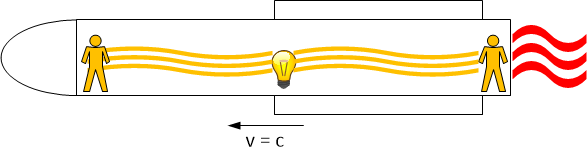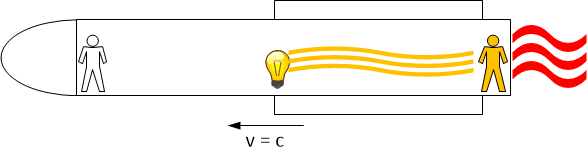# Inertial Observers: Galileo and Lorentz transformations

### Basic Concepts and principles

Two observers B and C are said to be inertial if they use inertial coordinates systems, ie between these systems, there are no movement or any relative moviment between them is rectilinear and uniform.

So to convert coordinates from one to another, simply add or subtract speeds, this is called the Galileo transformation.From the classical point of view to change coordinates between inertial systems, it is enough to add or subtract relative speeds, this is the Galileo transformation.
For example, if we travel on a train at 50km/h and run into the train in the opposite direction at 25km/h, it is obvious that an observer located on the platform outside the train will measure that our net speed is

50-25 = 25km/h

This works very well for low speeds, but if we consider speeds close to that speed of light, the situation is not so obvious, as we will see in the following simple example.

Suppose a rocket travels at the speed of light, and in the center of the rocket we turn on a light bulb. Imagine that we have an observer at each end of the rocket, for each of them the light will reach them at a time t, the same for each one since the distance to the bulb is the same. Both observers light up, as shown in the figure:The light in the center of the rocket illuminates both observers.

On the other hand, if we consider an observer outside the rocket, from the classical point of view it only has to add speeds, for the observer at the rear of the rocket the light of the bulb has the opposite direction to the rocket's march, so

$$v = c + c = 2c$$

because the speed of the rocket adds to that of light. In contrast to the observer at the front of the rocket, its speed has the same direction to the light coming out of the bulb, therefore, the speed of light for it is

$$v = c - c = 0$$

so for an observer outside the rocket, the light never reaches the observer located in the front and will never see it illuminated as the figure shows.From the classical point of view, for an observer outside the rocket the light could not reach the observer at the front of the rocket.

Note that in addition to the change of speeds for one and the other observer, we are talking about something deeper, because there is an absolute fact for the observer at the front of the rocket Does he light up? Does he illuminated from the bulb?

For situations like this, classical mechanics do not work. A new theory had to be created, where simultaneity will be relative to the observer and there not exists absolute concept for time or space. This is one of the central ideas of the Special Relativity of which Einstein and others knew how to seeº.

### Lorentz transformation

Suposse two intertial observers A and B who use (x, y, x, t) y (x', y', z', t') coordinates respectively. Suposse B moving by the x-axis of A at velocity constant v, ie for A

$\vec{v} = (v, 0, 0)$B reference system is moving in growing X-axis direction of A reference System.

Both systems are related by the Galilean Transformation
The transformation of Galileo
$$x'=x-vt$$ $$y'=y$$ $$z'=z$$ $$t'=t$$
We saw at section Maxwell equations that such equations may not hold for B especially if v is next to the value c = 300.000 km/s (aprox.).

Lorentz idea was study transformations that leave invariant Maxwell's equations, and concluded that the relation between both coordinates systems would be
The transformation of Lorentz
$$x'= \gamma(x -vt)$$ $$y'=y$$ $$z'=z$$ $$t'= \gamma(t -\frac{vx}{c^2})$$
Where
$$\gamma= \sqrt{1-\frac{v^2}{c^2}}$$

This is the Lorentz Transformation, it is essential to understand special relativity theory and as we will see, from this transformation emerge all relativity revolutionary consequences. Note that for speeds which we are accustomed to, the value γ is nearly 1 and the Lorentz transformation is nearly to be identical to the Galilean transformation.

A first, important observation is that to pass from one reference system to another, we must also transform time coordinate. Ie time is relative and depends on the reference system the observer were using to measure.

We will see finally a theorem that explains how to transform electromagnetic fields for inertial observers, such as for them all, Maxwell's equations holdss:

Consider two inertial observers A and B as before A moves to B with velocity

$\vec{v} = (v, 0, 0)$

. Suppose A uses the coordinate system (x, y, x, t) and B uses (x ', y', z ', t').

suposse also, that both coordinate systems are related by the Lorentz transformation we have seen before then.

Let $\vec{B}, \vec{B'}, \vec{E}, \vec{E'}$ vectorial functions related by the formulas

$E_1'=E_1$

$E_2'= \gamma(E_2-vB_3)$

$E_3'= \gamma(E_3+vB_2)$

$B_1'=B_1$

$B_2'= \gamma(B_2+v \frac{E_3}{c^2})$

$B_3'= \gamma(B_3-v \frac{E_2}{c^2})$

Then if $\vec{B}, \vec{E}$ hods the Maxwell's equations with the coordinates system of A, then $\vec{B'}, \vec{E'}$ hods Maxwell's equations with the system used by B
This theorem shows that if you use the Lorentz transformation to change coordinates between inertial reference systems, Maxwell's equations are true for all them.

## Was useful? want add anything?

Post here

### Post from other users

Post here